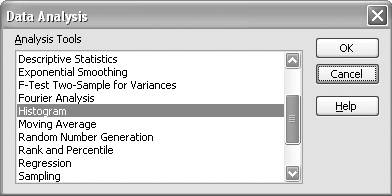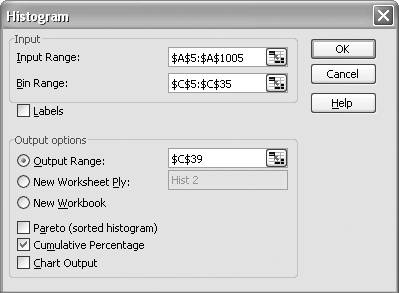# Plotting Frequency Distributions

Problem

You're analyzing a dataset and would like to create a histogram plot of the data showing its frequency distribution.

Solution

Use Excel's FREQUENCY function to compute the frequency of occurrence of each data point and use Excel's charting features (see Chapter 4) to create the histogram plot. Or use the Histogram tool in the Data Analysis add-in to compute the frequencies of occurrence and then use Excel's plotting features.

Discussion

Figure 5-4 shows a histogram plot (bar graph) along with a frequency polygon (the thin line) and cumulative frequency plot (the bold line) for a set of data. The dataset for this example plot represents the angular response of a torsional spring.

You can quickly create this sort of chart using the techniques discussed throughout Chapter 4. You'll first have to compute the actual frequency distribution for your dataset, and to that end you have two choices in Excel: the FREQUENCY function or the Histogram tool included in the Data Analysis add-in.

Figure 5-5 shows a portion of a spreadsheet containing the data used for the histogram plot shown in Figure 5-4.

Figure 5-4. Histogram plotThe angular response data contained in column A is the original dataset for which frequencies are computed. Before applying the FREQUENCY function, you must set up a range of discrete bin values, called bins. The bins form bands, or ranges of values, within which values in the original dataset will be lumped when compiling frequencies of occurrence. In this example, I know the angular response values fall within approximately -15 to 15 degrees; therefore, I set up a range of bin values from -15 to 15 degrees in increments of 1 degree. These are contained in column C in Figure 5-5.

To compute the frequencies for each bin, I applied the FREQUENCY formula, passing the dataset cell range as the first parameter and the bin cell range as the second parameter. For example, the formula in cell D5 is {=FREQUENCY(\$A\$5:\$A\$1005,\$C\$5:\$C\$35)}. Notice that this is an array formula and to enter it you must first select the entire output cell range, cells D5 to D35 in this case, and then enter the formula by pressing Ctrl-Shift-Enter.

Figure 5-5. Histogram example dataYou can compute such a frequency distribution using the Histogram tool instead of the FREQUENCY function if you'd like. To do so, select Tools [Image_Link]/images/U2192.jpg border=0> Data Analysis from the main menu bar to open the Data Analysis dialog box shown in Figure 5-6.

Then select the Histogram tool from the list of available tools and press OK to open the Histogram dialog box shown in Figure 5-7.

Figure 5-6. Data Analysis dialog boxFigure 5-7. Histogram tool dialog boxIn the Input Range field, enter or select from your spreadsheet the range of cells containing the input dataset. In the Bin Range field, enter or select the range of cells containing the bin values.

Under "Output options," select your preferred location for the output. In Figure 5-7, I set the output range to start at cell C39 in the same worksheet that contains the original dataset.

There are a few other options you can choose, and in this example I chose the Cumulative Percentage option to generate values for the cumulative plot shown earlier in Figure 5-4. Upon pressing OK, you should see results similar to those shown in Figure 5-5.Excel Scientific and Engineering Cookbook (Cookbooks (OReilly))
ISBN: 0596008791
EAN: 2147483647
Year: N/A
Pages: 206
Authors: David M Bourg